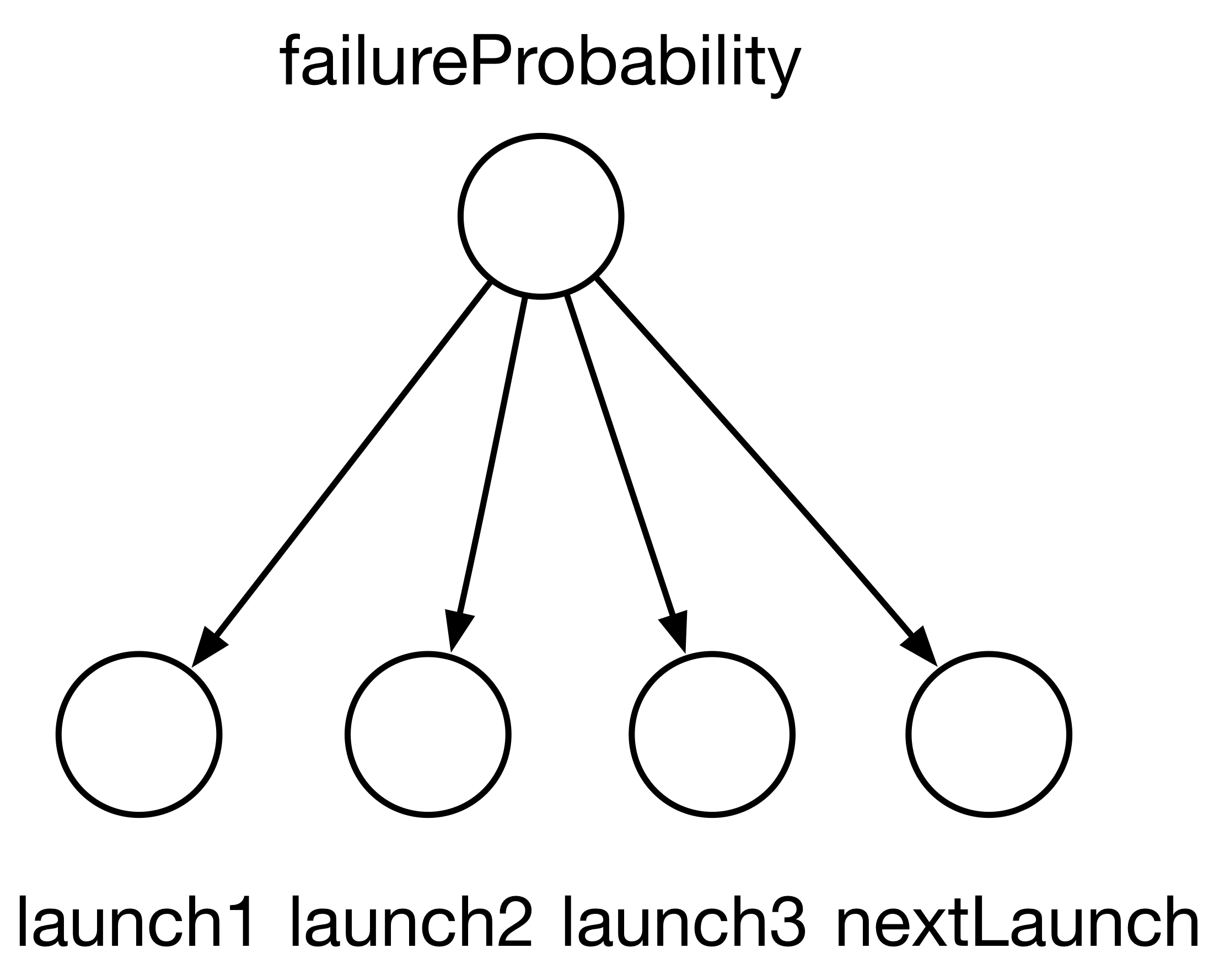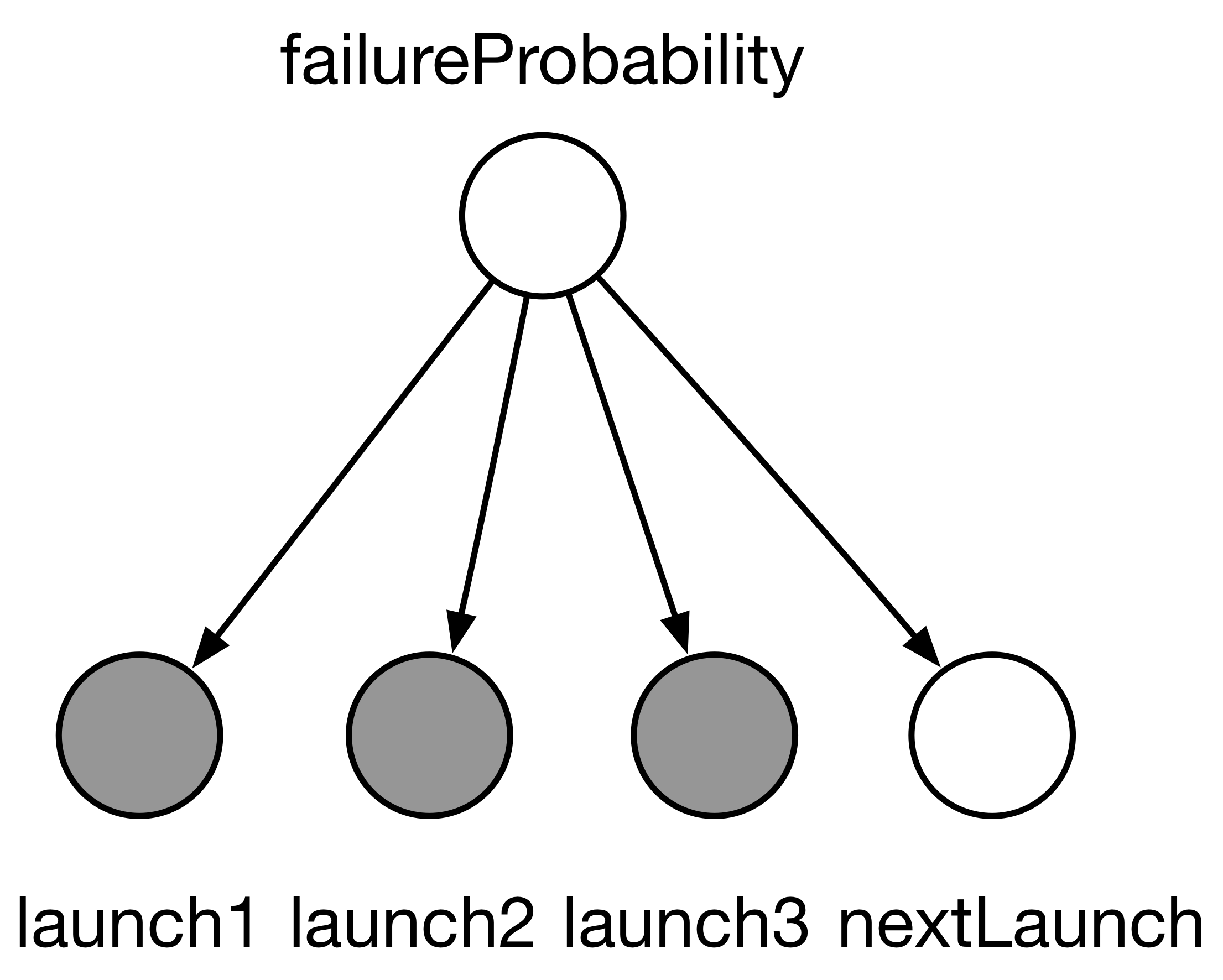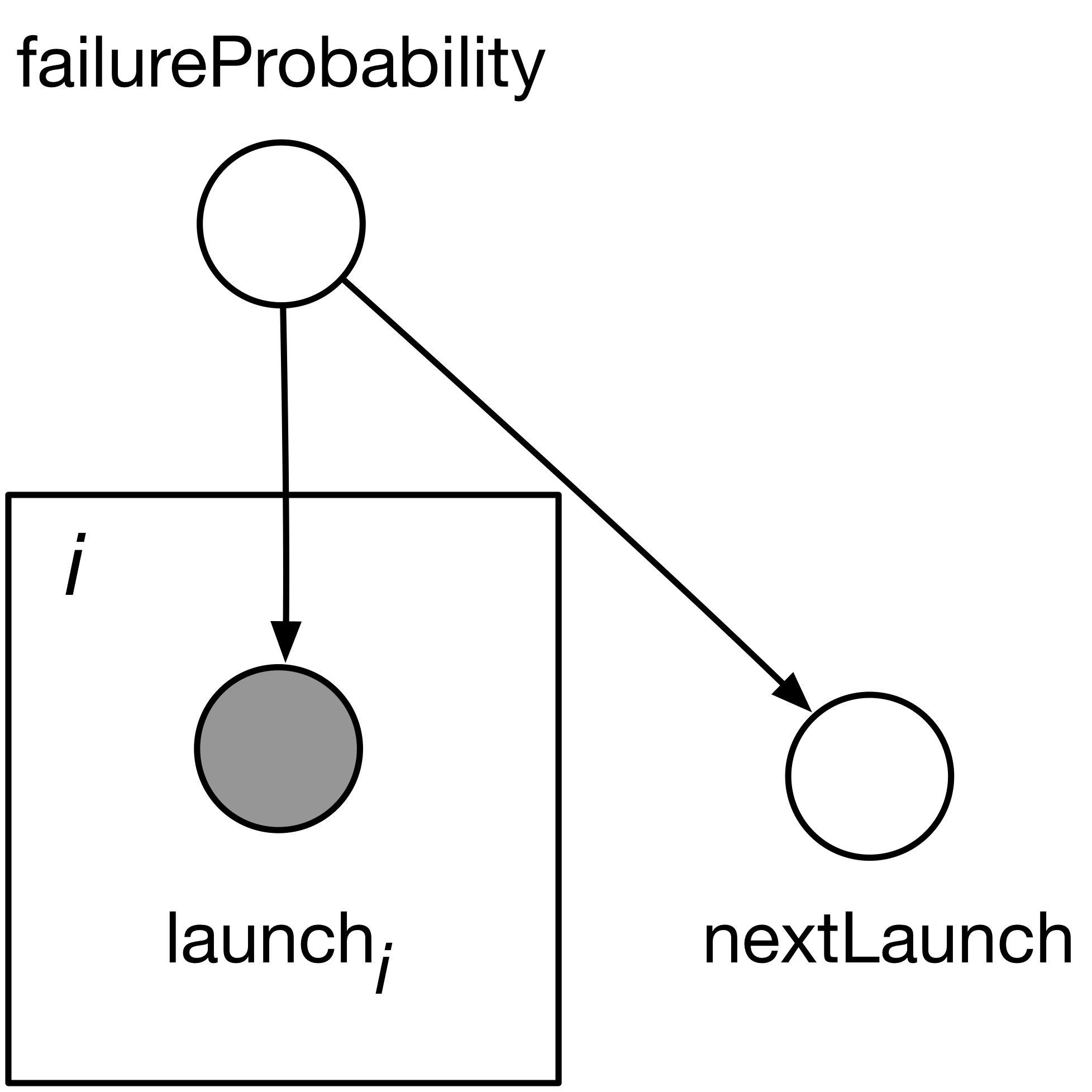# Graphical models

• The next few topics will be an overview of several useful Bayesian models (joint distributions over knowns and unknowns)
• Graphical models are pictures that will help you visualize Bayesian models
• There are several kinds of graphical models (directed, undirected, factor graphs)
• Today we will talk about directed graphical models

# Motivation: “generating data”

• “Generating data”: creating a fake dataset (also known as “synthetic data”)
• same data types as the real data, but different values
• Why generating data is useful:
• Testing inference procedures (both mathematical derivations and software implementation)
• Conceptual: in the Bayesian framework “model” $$=$$ “algorithm to generate data”
• The core data-structure needed to generate data is a directed graphical model

# Example

Recall the Delta rocket example:

launch1 | failureProbability ~ Bernoulli(failureProbability)
launch2 | failureProbability ~ Bernoulli(failureProbability)
launch3 | failureProbability ~ Bernoulli(failureProbability)

nextLaunch | failureProbability ~ Bernoulli(failureProbability)

failureProbability ~ ContinuousUniform(0, 1)

Suppose you want to generate a synthetic dataset.

### Poll: what order is most convenient to generate the 5 variables?

1. launch1, launch2, launch3, nextLaunch, failureProbability
2. failureProbability, launch1, launch2, launch3, nextLaunch
3. nextLaunch, failureProbability, launch1, launch2, launch3
4. nextLaunch, launch1, launch2, launch3, failureProbability
5. failureProbability, nextLaunch, launch1, launch2, launch3

# Example, continued

launch1 | failureProbability ~ Bernoulli(failureProbability)
launch2 | failureProbability ~ Bernoulli(failureProbability)
launch3 | failureProbability ~ Bernoulli(failureProbability)

nextLaunch | failureProbability ~ Bernoulli(failureProbability)

failureProbability ~ ContinuousUniform(0, 1)• Any order that starts with failureProbability is easy to implement (other orders all technically possible since chain rule applies in any order, but computationally more challenging as the conditional probabilities are then not directly available)
• How to generalize? Directed graphical model.
• Directed graphical model: a discrete directed graph where
• each vertex is a random variable from the model
• draw an edge x $$\to$$ y whenever the model description contains:
y | ..., x, ...
• Any ordering of the variables respecting the directionality of the graphical model’s edges yields an efficient algorithm for synthetic data generation
• “respecting the directionality of the edges”: technical definition is called “linearization of partial order”
• finding a linearization can be done in linear time using topological sorting

# Conditioning

Bonus: the notation can be used to represent a conditional distribution.

Convention: shade in gray the nodes we condition on (observations)# Plate notation

Plate: When there are too many vertices to draw in a graphical model, use a square (“plate”) to indicate a group of nodes that are repeated several times. A graphical “for loop”.# Further pointers on graphical models

Directed graphical models are also useful to identify conditional independence relationships (recall, $$A$$ is conditionally independent of $$B$$ give $$C$$ if $${\mathbb{P}}(A|C) {\mathbb{P}}(B|C) = {\mathbb{P}}(A \cap B | C)$$).

Watch this video to see how this is done, or read the original paper.